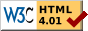## Dokumentation zu: first_inventory(E)``` SYNOPSIS object first_inventory() object first_inventory(string ob) object first_inventory(object ob) DESCRIPTION Get the first object in the inventory of ob, where ob is either an object or the file name of an object. If ob is not given, the current object is assumed. EXAMPLES This efun is mostly used in the following context: for(ob=first_inventory(container);ob;ob=next_inventory(ob)) { } If you use such calls frequently then it would be very useful to use a preprocessor macro: #define FORALL(x, y) for(x=first_inventory(y);x;x=next_inventory(x)) So the above example could be written like this: FORALL(ob, container) { } Warning: If the object ob is moved inside , then next_inventory() will return an object from the new inventory of ob. You also shouldn't call next_inventory() on destructed objects. So in case of move and/or destruction the following is a better solution: for(ob=first_inventory(container);ob;) { next=next_inventory(ob); ob=next; } SEE ALSO next_inventory(E), all_inventory(E), environment(E), deep_inventory(E) ``` Die Seite ist auch in Deutsch vorhanden.

Start » Magierhandbuch » Docu » Efun » First_inventory Letzte Generierung: 25.04.2021, 01:58mud@wl.mud.de